# Solutions MCQ - 1 (Advanced)

## 25 Questions MCQ Test Chemistry for JEE Advanced | Solutions MCQ - 1 (Advanced)

Description
This mock test of Solutions MCQ - 1 (Advanced) for JEE helps you for every JEE entrance exam. This contains 25 Multiple Choice Questions for JEE Solutions MCQ - 1 (Advanced) (mcq) to study with solutions a complete question bank. The solved questions answers in this Solutions MCQ - 1 (Advanced) quiz give you a good mix of easy questions and tough questions. JEE students definitely take this Solutions MCQ - 1 (Advanced) exercise for a better result in the exam. You can find other Solutions MCQ - 1 (Advanced) extra questions, long questions & short questions for JEE on EduRev as well by searching above.
QUESTION: 1

### At 300 K, the vapour pressure of an ideal solution containing 3 mole of A and 2 mole of B is 600 torr. At the same temperature, if 1.5 mole of A & 0.5 mole of C(non-volatile) are added to this solution the vapour pressure of solution increases by 30 torr. What is the value of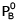?

Solution:

Initially A = 3 mole ; B = 2 mole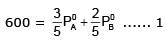finally A= 4.5 mole ; B = 2 mole and c = 0.5 mole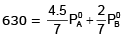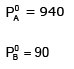QUESTION: 2

### The following graph represents variation of boiling point with composition of liquid and vapours of binary liquid mixture. The graph is plotted at constant pressure. Which of the following statement is incorrect. Here X & Y stands for mole fraction in liquid and vapour phase respectively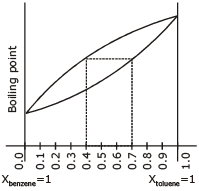Solution:

B.PTolulene > B.P.Benzene
V.P.Tolene < V.P.Benzene
Benzene is more volatile (By graph)
xBenzene < yBenzene
(A) If xB = 0.5 ⇒ yt = 0.2, yB = 0.8
(B) xt = 0.3, ⇒ xB = 0.7 ⇒ yB = 0.6 not possible
(C) xB = 0.3 and yt = 0.4
⇒ yB = 0.6  correct
(D) xB = 0.7. yB > 0.7 (should be)
Given yt < 0.3 ⇒ yB > 0.7 correct

QUESTION: 3

### The freezing point depression of a 0.1 M aq. solution of weak acid (HX) is – 0.20ºc. What is the value of equilibrium constant for the reaction? HX(aq)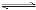H+(aq) + X_(aq) [Given : Kf for water 1.8 kg mol-1 K. & Molality = Molarity]

Solution:

0.20 = 1.8 × M × i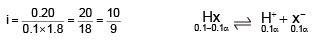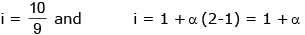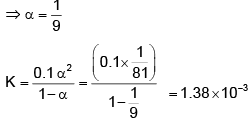QUESTION: 4

The van't Hoff factor for 0.1 M Ba(NO3)2 solution is 2.74. The degree of dissociation is

Solution:

i = 1 + α(n - 1)
2.74 = 1 + α(3 - 1)
⇒ α = 0.87
⇒ Degree of dissociation = 87%

QUESTION: 5

The vapour pressure of an aqueous solution is found to be 750 torr at certain temperature `T'. If `T' is the temperature at which pure water boils under atmospheric pressure and same solution show elevation in boiling point ΔTb = 1.04 K, find the atmospheric pressure. (Kb = 0.52 K kg mol-1)

Solution:

1.04 = 0.52 × m ⇒ m = 2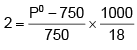⇒ P0 = 777 = Patm
P0 = Patm (because at T water boils)
So at T : P0 = Patm
because when water boils V.P. become equals to atmospheric pressure

*Multiple options can be correct
QUESTION: 6

Study the following figure and choose the correct options from the given below (assuming both dissociate completely).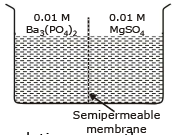Solution:

A There will be movement of solvent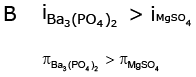C Solvent flow from right (MgSO4) to left (Ba3(PO4)2)
D No flow of solute
⇒ no ppt formation

*Multiple options can be correct
QUESTION: 7

When CuSO4 is dissolved in NH4OH solution then the correct statement is

Solution: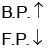*Multiple options can be correct
QUESTION: 8

The examples of negative deviation are

Solution:

polar - polar → -ve deviation

*Multiple options can be correct
QUESTION: 9

Acetone and carbon disulphide form binary liquid solution showing positive deviation from Raolut law. The normal polling point (Tb) of pure acetone is less than that of pure CS2. Pick out the incorrect statements among the following.

Solution:
*Multiple options can be correct
QUESTION: 10

Passage

Vapour pressure of a solvent is the pressure exerted by vapours when they are in equilibrium with its solvent at that temperature. The vapour pressure of solvent is dependent on nature of  solvent, temperature, addition of non-volatile solute as well as nature of solute to dissociate or associate.
The vapur pressuere  of a mixture obtained by mixing two volatile liquids is given by PM =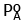. XA +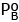. XB whereandare vapour pressures of  pure components A and B and XA, XB are their  mole fraction in mixture . For solute-solvent system, the realtion become PM =. XA where  B is non-volatile solute.

Q.

The vapour pressure of benzene and its solution with a non-electrolyte are 640 and 600 mm respectively. The molality of the solution is -

Solution: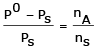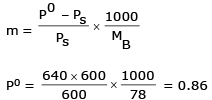QUESTION: 11

Vapour pressure of a solvent is the pressure exerted by vapours when they are in equilibrium with its solvent at that temperature. The vapour pressure of solvent is dependent on nature of  solvent, temperature, addition of non-volatile solute as well as nature of solute to dissociate or associate.
The vapur pressuere  of a mixture obtained by mixing two volatile liquids is given by PM =. XA +. XB whereandare vapour pressures of  pure components A and B and XA, XB are their  mole fraction in mixture . For solute-solvent system, the realtion become PM =. XA where  B is non-volatile solute.

Q.

A mixture of two volatile liquids A and B for 1 and 3 moles respectivley has a V.P. of 300 mm at 27ºC. If one mole of A is further added to this solution, the vapour pressure becomes 290 mm at 27ºC. The vapour pressure of pure A is -

Solution: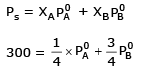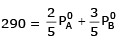P0 = 250

QUESTION: 12

Vapour pressure of a solvent is the pressure exerted by vapours when they are in equilibrium with its solvent at that temperature. The vapour pressure of solvent is dependent on nature of  solvent, temperature, addition of non-volatile solute as well as nature of solute to dissociate or associate.
The vapur pressuere  of a mixture obtained by mixing two volatile liquids is given by PM =. XA +. XB whereandare vapour pressures of  pure components A and B and XA, XB are their  mole fraction in mixture . For solute-solvent system, the realtion become PM =. XA where  B is non-volatile solute.

Q.

The amount of solute (mol. wt. 60) required to dissolve in 180 g of water to reduce the vapour pressure to 4/5 of the pure water -

Solution: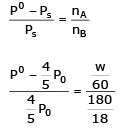w = 150 gm

QUESTION: 13

Statement - I. Addition of a non-volatile solute causes a depression in vapour pressure.

Statement - II. Vapour pressure of a solution is directly proportional to mole fraction of solvent.

Solution:

Statement-1 addition of nonvolatile solute causes a depression in v.p.
Statement-2 It is also correct because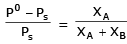so, it is directly proportional to the mole fraction of solute

QUESTION: 14

Statement - I. 0.02 m solutions of urea and sucrose will freeze at same temperature.

Statement - II. Freezing point of a solution is inversely proportional to the concentration of solution.

Solution:

Statement 1- It is correct because both are non electrolyte so both have same particle so ΔTf will be same.
Statement 2- ΔTf  = Kf × m
It is also correct because on increasing m ΔTf  will increase and freezing point will decrease but not the correct explanation of I.

QUESTION: 15

Statement - I. For isotonic solutions C1 = C2

Statement - II. For isotonic solutions p1 = p2

Solution:

For isotonic stution
i1C1 = i2C2 so statement I is wrong but for isotonic solution
π1 = π2
So statement II is wright

QUESTION: 16

Statement - I. Benzene-toluene mixture forms ideal solution.

Statement - II. Components with structural similarity forms ideal solution in general.

Solution:

Dilute solution shown ideal behaviors so statment I is wright and components with structural similarity froms ideal solution so statment I is wright explantion of II

QUESTION: 17

Statement - I. Dissolution of sulphuric acid in water gives a solution which shows negative deviation.

Statement - II. The solutions which have same vapour pressure at a given temperature are called isotonic solutions.

Solution:

Statement I is Correct because dissolution ion dipole interaction will make it to show negative deviation.
Statement II is wrong because solution having same osmotic pressure because is called isotonic solution.

QUESTION: 18

Statement - I. Greater the molal depression constant of the solvent used less in the freezing point of the solution.

Statement - II. Depression in freezing point depends upon the nature of the solvent

Solution:

Statement-1 is correct and statement II is also wrigth because depression in freezing bond depenels upon the nature of the solvent and is also the correct explanation.

QUESTION: 19

Column I

(A) Ideal solution

(B) Solutions showing positive deviations

(C) Solutions showing negative deviations

Column II

(P) Solute-solvent interactions are weaker than solute-solute

(Q) Solute-solvent interactions are similar to solute-solute

(R) Solute-solvent interactions are stronger than solute-solute interactions

Solution:

where solute-solvent  interaction solute-solvent interaction. then ideal solution.
B– solute-solvent interaction are weaker than solute-solvent so it shows positive deviation.
C– solute-solvent interactions stronger than solute-solvent  interaction it will show negative deviation.

QUESTION: 20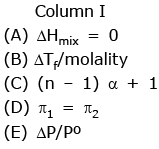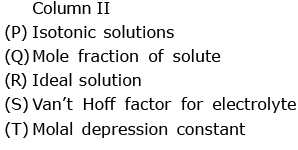Solution:
*Answer can only contain numeric values
QUESTION: 21

At 25ºC, the vapour pressure of methyl alcohol is 96.0 torr. What is the mole fraction of CH3OH in a solution in which the (partial) vapour pressure of CH3OH is 23.0 torr at 25ºC?

Solution: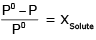CH3OH : Solvent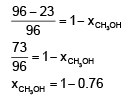= 0.24

*Answer can only contain numeric values
QUESTION: 22

The vapour pressure of pure liquid solvent A is 0.80 atm. When a non-volatile substance B is added to the solvent, its vapour pressure drops to 0.60 atm.what is the mole fraction of the component B in the solution ?

Solution: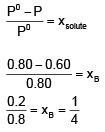*Answer can only contain numeric values
QUESTION: 23

The vapour pressure of pure water at 26ºC is 25.21 torr. What is the vapour pressure of a solution which contains 20.0 glucose, C6H12O6, in 70 g water?

Solution: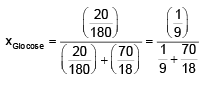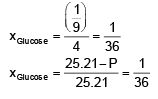⇒ 25.21 = 25.21 × 36 - P × 36
⇒ P = 24.5

*Answer can only contain numeric values
QUESTION: 24

The vapour pressure of pure water at 25ºC is 23.76 torr. The vapour pressure of a solution containing 5.40 g of a nonvolatile substance in 90.0 g water is 23.32 torr. Compute the molecular weight of the solute.

Solution: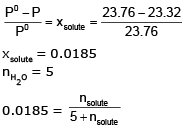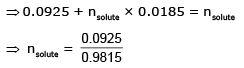nsolute = 0.094
Msolute = 57.4

*Answer can only contain numeric values
QUESTION: 25

The vapour pressure of ethanol and methanol are 44.5 mm and 88.7 mm Hg respectively. An ideal solution is prepared at the same temperature by mixing 60 g of ethanol with 40 g of methanol. Calculate total vapour pressure of the solution.

Solution: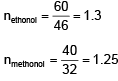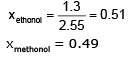P = 0.51 × 44.5 + 0.49 × 88.7
= 66.15